• 如果要把unity3D的工程，转到flash平台，是有一些限制的，其中，unity3d地形不能使用，这就是一个很大的问题。 所以，使用mesh来替代unity自带的地形，就变成首选的解决方案。 问题接着而来，mesh怎么像unity...
如果要把unity3D的工程，转到flash平台，是有一些限制的，其中，unity3d的地形不能使用，这就是一个很大的问题。
所以，使用mesh来替代unity自带的地形，就变成首选的解决方案。
问题接着而来，mesh怎么像unity自带的地形一样刷地表贴图，怎么种树，草。这就需要一套完整的系统与工具来支持。
于是有了这个新的工具。
half4 splat_control = tex2D (_Control, IN.uv_Control);half3 col;col  = splat_control.r * tex2D (_Splat0, IN.uv_Splat0).rgb;col += splat_control.g * tex2D (_Splat1, IN.uv_Splat1).rgb;col += splat_control.b * tex2D (_Splat2, IN.uv_Splat2).rgb;col += splat_control.a * tex2D (_Splat3, IN.uv_Splat3).rgb;上图中，scene窗口中，原点就是笔刷，笔刷可以预览选中的贴图，方便美术绘制。
2 种树，这个就更简单了，把树的模型放到mesh表面。


展开全文flash Flash Unity3D unity3d
• Unity3D中的地形转成模型 浪尖儿关注 2016.08.17 17:02*字数 569阅读 5029评论 0喜欢 9 Unity3D中的地形转成模型 起因 为什么要把地形转成模型呢？在Unity3D中创建地形很方便，用它自带的地形编辑工具，各种...
Unity3D中的地形转成模型浪尖儿 关注

2016.08.17 17:02* 字数 569 阅读 5029评论 0喜欢 9

Unity3D中的地形转成模型

起因

为什么要把地形转成模型呢？在Unity3D中创建地形很方便，用它自带的地形编辑工具，各种跌宕起伏的地形都能很容易的创建出来。但是也有一些不方便的地方，比如创建好的地形不能整体缩放，只能通过修改长宽等参数进行调整。偏偏就有这样的需求，我们要把地形放到虚拟研讨厅的桌子上当做数字沙盘去展示。如果把地形对象的长宽高都缩小的话，高度图也要缩小，地形效果就太不好了。如果地形对象能像模型对象一样，随意的缩放就好了。

解决方法

终于在网上找到了解决方法，有大神贡献了一个脚本，能够把地形对象转换成模型对象，模型格式为obj，可以直接导入到Unity3D中使用。

using UnityEngine;
using UnityEditor;
using System;
using System.Collections;
using System.IO;using System.Text;

enum SaveFormat { Triangles, Quads }
enum SaveResolution { Full=0, Half, Quarter, Eighth, Sixteenth }

class ExportTerrain : EditorWindow
{
SaveFormat saveFormat = SaveFormat.Triangles;
SaveResolution saveResolution = SaveResolution.Half;

static TerrainData terrain;
static Vector3 terrainPos;

int tCount;
int counter;
int totalCount;
int progressUpdateInterval = 10000;

static void Init()
{
terrain = null;
Terrain terrainObject = Selection.activeObject as Terrain;
if (!terrainObject)
{
terrainObject = Terrain.activeTerrain;
}
if (terrainObject)
{
terrain = terrainObject.terrainData;
terrainPos = terrainObject.transform.position;
}

EditorWindow.GetWindow<ExportTerrain>().Show();
}

void OnGUI()
{
if (!terrain)
{
GUILayout.Label("No terrain found");
if (GUILayout.Button("Cancel"))
{
EditorWindow.GetWindow<ExportTerrain>().Close();
}
return;
}
saveFormat = (SaveFormat) EditorGUILayout.EnumPopup("Export Format", saveFormat);

saveResolution = (SaveResolution) EditorGUILayout.EnumPopup("Resolution", saveResolution);

if (GUILayout.Button("Export"))
{
Export();
}
}

void Export()
{
string fileName = EditorUtility.SaveFilePanel("Export .obj file", "", "Terrain", "obj");
int w = terrain.heightmapWidth;
int h = terrain.heightmapHeight;
Vector3 meshScale = terrain.size;
int tRes = (int)Mathf.Pow(2, (int)saveResolution );
meshScale = new Vector3(meshScale.x / (w - 1) * tRes, meshScale.y, meshScale.z / (h - 1) * tRes);
Vector2 uvScale = new Vector2(1.0f / (w - 1), 1.0f / (h - 1));
float[,] tData = terrain.GetHeights(0, 0, w, h);

w = (w - 1) / tRes + 1;
h = (h - 1) / tRes + 1;
Vector3[] tVertices = new Vector3[w * h];
Vector2[] tUV = new Vector2[w * h];

int[] tPolys;

if (saveFormat == SaveFormat.Triangles)
{
tPolys = new int[(w - 1) * (h - 1) * 6];
}
else
{
tPolys = new int[(w - 1) * (h - 1) * 4];
}

// Build vertices and UVs
for (int y = 0; y < h; y++)
{
for (int x = 0; x < w; x++)
{
tVertices[y * w + x] = Vector3.Scale(meshScale, new Vector3(-y, tData[x * tRes, y * tRes], x)) + terrainPos;
tUV[y * w + x] = Vector2.Scale( new Vector2(x * tRes, y * tRes), uvScale);
}
}

int  index = 0;
if (saveFormat == SaveFormat.Triangles)
{
// Build triangle indices: 3 indices into vertex array for each triangle
for (int y = 0; y < h - 1; y++)
{
for (int x = 0; x < w - 1; x++)
{
// For each grid cell output two triangles
tPolys[index++] = (y * w) + x;
tPolys[index++] = ((y + 1) * w) + x;
tPolys[index++] = (y * w) + x + 1;

tPolys[index++] = ((y + 1) * w) + x;
tPolys[index++] = ((y + 1) * w) + x + 1;
tPolys[index++] = (y * w) + x + 1;
}
}
}
else
{
// Build quad indices: 4 indices into vertex array for each quad
for (int y = 0; y < h - 1; y++)
{
for (int x = 0; x < w - 1; x++)
{
// For each grid cell output one quad
tPolys[index++] = (y * w) + x;
tPolys[index++] = ((y + 1) * w) + x;
tPolys[index++] = ((y + 1) * w) + x + 1;
tPolys[index++] = (y * w) + x + 1;
}
}
}

// Export to .obj
StreamWriter sw = new StreamWriter(fileName);
try
{

sw.WriteLine("# Unity terrain OBJ File");

// Write vertices
counter = tCount = 0;
totalCount = (tVertices.Length * 2 + (saveFormat == SaveFormat.Triangles ? tPolys.Length / 3 : tPolys.Length / 4)) / progressUpdateInterval;
for (int i = 0; i < tVertices.Length; i++)
{
UpdateProgress();
StringBuilder sb = new StringBuilder("v ", 20);
// StringBuilder stuff is done this way because it's faster than using the "{0} {1} {2}"etc. format
// Which is important when you're exporting huge terrains.
sb.Append(tVertices[i].x.ToString()).Append(" ").
Append(tVertices[i].y.ToString()).Append(" ").
Append(tVertices[i].z.ToString());
sw.WriteLine(sb);
}
// Write UVs
for (int i = 0; i < tUV.Length; i++)
{
UpdateProgress();
StringBuilder sb = new StringBuilder("vt ", 22);
sb.Append(tUV[i].x.ToString()).Append(" ").
Append(tUV[i].y.ToString());
sw.WriteLine(sb);
}
if (saveFormat == SaveFormat.Triangles)
{
// Write triangles
for (int i = 0; i < tPolys.Length; i += 3)
{
UpdateProgress();
StringBuilder sb = new StringBuilder("f ", 43);
sb.Append(tPolys[i] + 1).Append("/").Append(tPolys[i] + 1).Append(" ").
Append(tPolys[i + 1] + 1).Append("/").Append(tPolys[i + 1] + 1).Append(" ").
Append(tPolys[i + 2] + 1).Append("/").Append(tPolys[i + 2] + 1);
sw.WriteLine(sb);
}
}
else
{
for (int i = 0; i < tPolys.Length; i += 4)
{
UpdateProgress();
StringBuilder sb = new StringBuilder("f ", 57);
sb.Append(tPolys[i] + 1).Append("/").Append(tPolys[i] + 1).Append(" ").
Append(tPolys[i + 1] + 1).Append("/").Append(tPolys[i + 1] + 1).Append(" ").
Append(tPolys[i + 2] + 1).Append("/").Append(tPolys[i + 2] + 1).Append(" ").
Append(tPolys[i + 3] + 1).Append("/").Append(tPolys[i + 3] + 1);
sw.WriteLine(sb);
}
}
}
catch(Exception err)
{
Debug.Log("Error saving file: " + err.Message);
}
sw.Close();

terrain = null;
EditorUtility.DisplayProgressBar("Saving file to disc.", "This might take a while...", 1f);
EditorWindow.GetWindow<ExportTerrain>().Close();
EditorUtility.ClearProgressBar();
}

void UpdateProgress()
{
if (counter++ == progressUpdateInterval)
{
counter = 0;
EditorUtility.DisplayProgressBar("Saving...", "", Mathf.InverseLerp(0, totalCount, ++tCount));
}
}
}


使用方法

给脚本命名为ExportTerrain.cs。
这个脚本文件一定要放到工程中Assets文件夹下面的Editor文件夹中（没有的话自己创建）才能正常工作。
完成前两步之后，unity菜单项会多出一个Terrain/Export To Obj...的菜单(4.3以前的版本本来就有Terrain菜单，只是多了个子菜单；4.3版本里面默认没有Terrain菜单了)。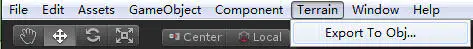选择场景要转成模型的地形对象。如果什么都没选的话，会使用默认的active terrain。

然后选择Terrain/Export To Obj...菜单，弹出下面的对话框，选择导出格式（triangles or quads）、Mesh分辨率（full, half, quarter, eighth or sixteenth）、文件名和路径，然后点击Export。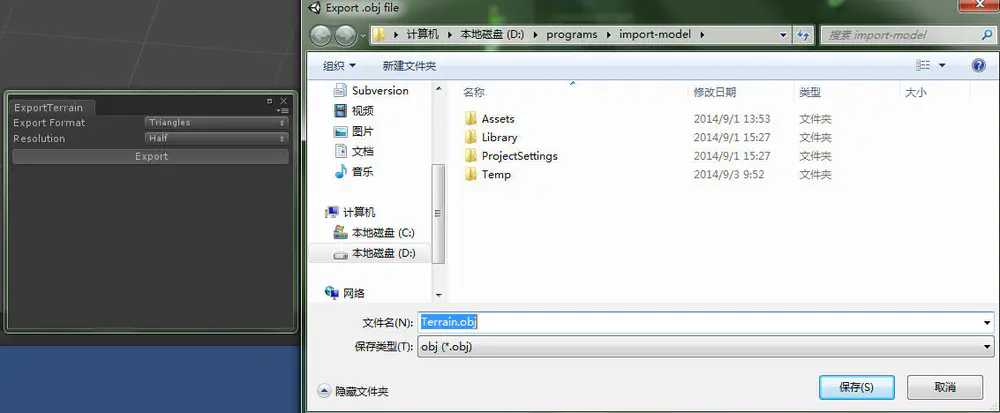terrain-expoty

等待进度条跑完之后就OK了，obj文件就导出成功了。

和地形转模型的方法相反的一个操作，还有模型转地形的方法，类似的也是有脚本完成的，感兴趣可以参考Object2Terrain.

参考

http://wiki.unity3d.com/index.php?title=TerrainObjExporterhttp://wiki.unity3d.com/index.php?title=Object2Terrain


展开全文• Unity 3D 创建Mesh 一、Unity 3D 创建面片（MeshMesh是一种网格，可以产生像地形那样震撼的效果，那么怎样创建Mesh呢？那就要知道Mesh包含什么！ Mesh(网格)：顶点、三角形、段数（一条直线有段）。 如图所示：...

Unity 3D 创建Mesh（一）

一、Unity 3D 创建面片（Mesh）
Mesh是一种网格，可以产生像地形那样震撼的效果，那么怎样创建Mesh呢？那就要知道Mesh包含什么！
Mesh(网格)：顶点、三角形、段数。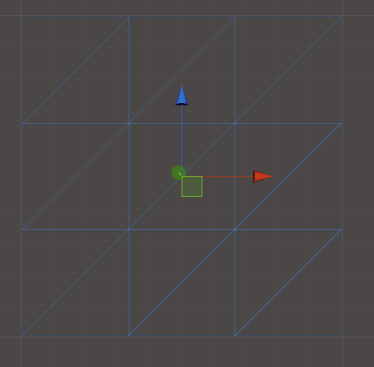如图所示：该网格该如何表示呢？

顶点（vertexes）
16=4*4
段数（segment）
3*3
三角形（triangles）
18

但是，三角形数目不是自己数，而是计算出来的。

二、创建Mesh属性
我们来创建一个长宽为100m*100m，高度为0m，段数为3*3的网格，如上图所示
/*  Mesh属性
*      长宽
*      段数
*      高度
*/
private Vector2 size;//长度和宽度
private float height= 0;//高度
private Vector2 segment;//长度的段数和宽度的段数/*  顶点属性
*      顶点
*      uv
*      三角形
*/
private Vector3[] vertexes;//顶点数
private int[] triangles;//三角形索引
    /*计算顶点，存入顶点数组*/    private void computeVertexes()
{
int sum = Mathf.FloorToInt((segment.x + 1) * (segment.y + 1));//顶点总数
float w = size.x / segment.x;//每一段的长度
float h = size.y / segment.y;

GetTriangles();//计算三角形索引顶点序列

int index = 0;
vertexes = new Vector3[sum];
for (int i = 0; i < segment.y + 1;i++ )
{
for (int j = 0; j < segment.x + 1; j++)
{
float tempHeight = 0;
vertexes[index] = new Vector3(j*w, 0, i*h);//计算完顶点
index++;
}
}
}/*计算三角形的顶点索引*/private int[] GetTriangles()
{
int sum = Mathf.FloorToInt(segment.x * segment.y * 6);//三角形顶点总数：假设是1*1的网格，会有2个顶点复用，因此是6个顶点。假设是2*2的网格，则是4个1*1的网格，即4*6即2*2*6！
triangles = new int[sum];
uint index = 0;
for (int i = 0; i < segment.y; i++)
{
for (int j = 0; j < segment.x; j++)
{
int role = Mathf.FloorToInt(segment.x) + 1;
int self = j + (i * role);
int next = j + ((i + 1) * role);
//顺时针//第一个三角形
triangles[index] = self;
triangles[index + 1] = next + 1;
triangles[index + 2] = self + 1;//第二个三角形
triangles[index + 3] = self;
triangles[index + 4] = next;
triangles[index + 5] = next + 1;
index += 6;
}
}
return triangles;
}

到此：顶点、三角形计算完成！接下来是渲染。
三、渲染网格
在Unity 3D中，一个GameObject只有一个transform组件。我们在程序中，声明一个GameObject变量，通过添加MeshFilter来增加Mesh属性，通过MeshRenderer渲染出来，在MeshRenderer中，我们使用默认材质球。
    private GameObject mMesh;
private Material mMaterial;mMesh = new GameObject();
mMesh.name = "CreateMesh";</pre><pre code_snippet_id="655703" snippet_file_name="blog_20150429_12_2096575" name="code" class="cpp">    private void DrawMesh()
{

mMesh.GetComponent<Renderer>().material = mMaterial;

/*设置mesh*/
mesh.Clear();//更新
mesh.vertices = vertexes;
//mesh.uv
mesh.triangles = triangles;

mesh.RecalculateNormals();
mesh.RecalculateBounds();

}

四、完整代码（有点不一样，扩充了点，拖在摄像机下面即可）

using UnityEngine;
using System.Collections;

/*仅仅创建Mesh
*
*
*/
public class CreatMesh : MonoBehaviour {

private GameObject mMesh;
private Material mMaterial;

/*  Mesh属性
*      长宽
*      段数
*      高度
*      高度差
*/
private Vector2 size;//长度和宽度
private float minHeight = -10;//最小高度
private float maxHeight = 10;//最大高度
private Vector2 segment;//长度的段数和宽度的段数
private float unitH;//最小高度和最大高度只差，值为正

/*  顶点属性
*      顶点
*      uv
*      三角形
*/
private Vector3[] vertexes;//顶点数
private Vector2 uvs;//uvs坐标
private int[] triangles;//三角形索引

void Start () {
creatMesh(100, 100, 3, 3, -10, 10);
}

private void creatMesh(float width, float height, uint segmentX, uint segmentY,int min, int max)
{
size = new Vector2(width, height);
maxHeight = max;
minHeight = min;
unitH = maxHeight - minHeight;
segment = new Vector2(segmentX, segmentY);

if (mMesh != null)
{
Destroy(mMesh);
}
mMesh = new GameObject();
mMesh.name = "CreateMesh";

computeVertexes();
DrawMesh();
}

private void computeVertexes()
{
int sum = Mathf.FloorToInt((segment.x + 1) * (segment.y + 1));//顶点总数
float w = size.x / segment.x;//每一段的长度
float h = size.y / segment.y;

GetTriangles();

int index = 0;
vertexes = new Vector3[sum];
for (int i = 0; i < segment.y + 1;i++ )
{
for (int j = 0; j < segment.x + 1; j++)
{
float tempHeight = 0;
vertexes[index] = new Vector3(j*w, 0, i*h);
index++;
}
}
}

private void DrawMesh()
{

mMesh.GetComponent<Renderer>().material = mMaterial;

/*设置mesh*/
mesh.Clear();//更新
mesh.vertices = vertexes;
//mesh.uv
mesh.triangles = triangles;

mesh.RecalculateNormals();
mesh.RecalculateBounds();

}

private int[] GetTriangles()
{
int sum = Mathf.FloorToInt(segment.x * segment.y * 6);//三角形顶点总数
triangles = new int[sum];
uint index = 0;
for (int i = 0; i < segment.y; i++)
{
for (int j = 0; j < segment.x; j++)
{
int role = Mathf.FloorToInt(segment.x) + 1;
int self = j + (i * role);
int next = j + ((i + 1) * role);
//顺时针
triangles[index] = self;
triangles[index + 1] = next + 1;
triangles[index + 2] = self + 1;
triangles[index + 3] = self;
triangles[index + 4] = next;
triangles[index + 5] = next + 1;
index += 6;
}
}
return triangles;
}
}



展开全文• 之前这个博客： ...主要是关于如何把场景中的物件，进行空间划分，并且根据主角的位置，动态的加载周边的物件，对于大地形的处理，则没有涉及到。 经过搜索，主要收集了如下具有参考价值的网址： ...
之前这个博客：
主要是关于如何把场景中的物件，进行空间划分，并且根据主角的位置，动态的加载周边的物件，对于大地形的处理，则没有涉及到。
经过搜索，主要收集了如下具有参考价值的网址：
重点参考三个网址：
http://www.cnblogs.com/jietian331/p/5831062.html 本文重点讲述
https://blog.csdn.net/zr339361504/article/details/53352800 这个文章涉及法线的计算
https://gitee.com/langresser_king/terrain_proj 码云有代码，分割地形的方法同上面一个网址
https://catlikecoding.com/unity/tutorials/procedural-grid/ 一个很好的关于unity学习的网址，后面会重点研究和这个博客
http://www.52vr.com/article-1173-1.html 无限大地形生成
http://c.biancheng.net/view/2739.html
https://www.bilibili.com/video/av4380122?from=search&seid=13314329861953277930 视频教程高度图生成地形
http://darrellbircsak.com/2017/01/27/split-unity-terrain-script/ 分割地形的blog
https://kostiantyn-dvornik.blogspot.com/2013/12/unity-split-terrain-script.html
https://unity3d.com/how-to/big-games-on-low-end-mobile
https://www.gamasutra.com/blogs/ChristophEnder/20170222/292179/Open_World_on_Mobile_with_Unity.php
https://github.com/tangrams/unity-terrain-example
https://docs.unity3d.com/ScriptReference/TerrainData.GetAlphamaps.html
https://www.jianshu.com/p/264e9665f6b1
https://www.bbsmax.com/A/amd0624LJg/
https://connect.unity.com/p/mte-mesh-terrain-editor-mo-xing-di-xing-bian-ji-qi
https://www.bilibili.com/video/av10191087/
https://www.bilibili.com/video/av10191087/?p=1 bili教程
http://new-play.tudou.com/v/XMjQ1MTY5MTY4MA==.html 土豆mte教程
http://www.vr2.tv/develop/unity-chajian-kaifa-jieshao.html 十款unity必备插件
https://gameinstitute.qq.com/community/detail/126192 lam地编工具
https://github.com/wachel/TerrainToLodMesh  github 将terrain转换mesh
https://github.com/jinsek/MightyTerrainMesh  四叉树加载mesh github源码
https://zhuanlan.zhihu.com/p/64809281 上面的知乎博客
https://zhuanlan.zhihu.com/p/53355843 知乎高度图生成terrain
https://www.cnblogs.com/AZ-ZK/p/4219981.html  感觉是个大牛
http://gulu-dev.com/ 感觉是个大牛
http://oldking.wang/ 隔壁老王的博客
关于插件：
t4m 过时
mte 只有dll
terrain to mesh 只有dll
上面的这几个也曾视图去分析是否源码，然后进行分析，定制自己的unity terrain转mesh的需求，但是未果，所以还是重点参考了两篇文章：
http://www.cnblogs.com/jietian331/p/5831062.html 本文重点讲述
https://gitee.com/langresser_king/terrain_proj 码云有代码，分割地形的方法同上面一个网址
ok，下面来重点分析下其实现的过程。
1、首先是把unity的地形转为mesh
要明白为啥这么做？
引用https://zhuanlan.zhihu.com/p/64809281的一段文字：

Unity的Terrain一直被移动开发团队诟病其可用性，使用原生的Terrain的移动项目大部分都是平地，仅使用了贴图混合的部分。对于有高低起伏的Terrain采用转为mesh的方式使用，早期常用的插件如T4M和Terrain2Mesh，在Unity2018对terrain做了改动以后貌似都不再更新了。T4M更倾向于一个方便Artist修改模型的工具，不过一个模型反反复复地在多个工具中来回实在是很痛苦。Terrain2Mesh使用的人不多，本身比较简单，仅能导出固定规则网格和材质，限制也比较多。

归结为一句话就是性能，unity提供的terrain性能较差。再加上如果是特大地形就必须分开加载，综上，需要将terrain转换为mesh，然后进行分块处理。
下面就是代码的部分，首先是进行terrain转mesh：

static void Init()
{
//1.所选择的物体是否为空，在Hierarchy视图选中一个物体即可。
if (Selection.objects.Length <= 0)
{
Debug.Log("Selection.objects.Length <= 0");
return;
}

var terrainObj = Selection.objects as GameObject;
if (terrainObj == null)
{
Debug.Log("terrainObj == null");
return;
}

//2.获取地形选中物体身上的组件Terrain
var terrain = terrainObj.GetComponent<Terrain>();
if (terrain == null)
{
Debug.Log("terrain == null");
return;
}
//3.判断是否有地形数据
var terrainData = terrain.terrainData;
if (terrainData == null)
{
Debug.Log("terrainData == null");
return;
}

下面看看unity给我们提供的地形的样子：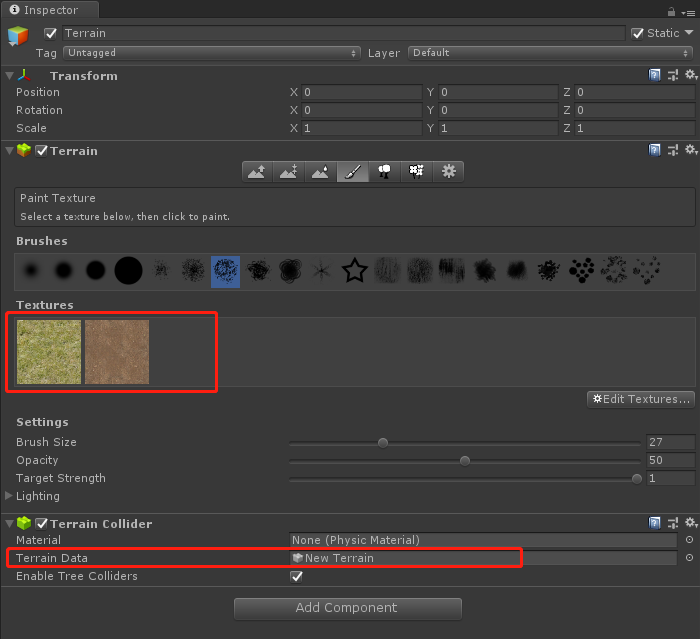int vertexCountScale = 4;
int w = terrainData.heightmapWidth; //高度图的宽度
int h = terrainData.heightmapHeight;//高度图的高度
Vector3 size = terrainData.size; //地形的长宽高

这里很多人会对接下来的代码产生怀疑，我也不例外，但是经过逐行的理解，还是参悟了其中的道理。
首先是vertexCountScale的意义，其实就是距离多远采样一个点，也就是采样的精度。
比如地形原先是10001000大小：
那么如果按照距离4进行采样，最后得出的就是250250个点。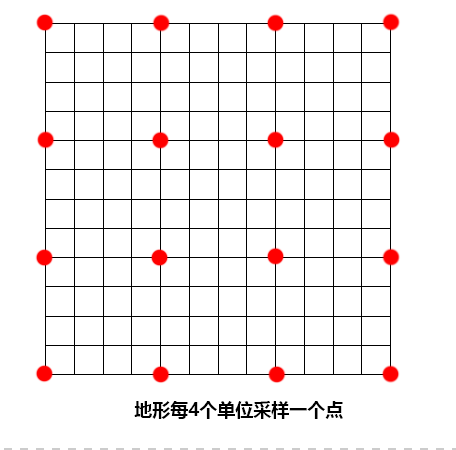高度图的宽和高，以及地形的长宽高，在配置中可以看出来：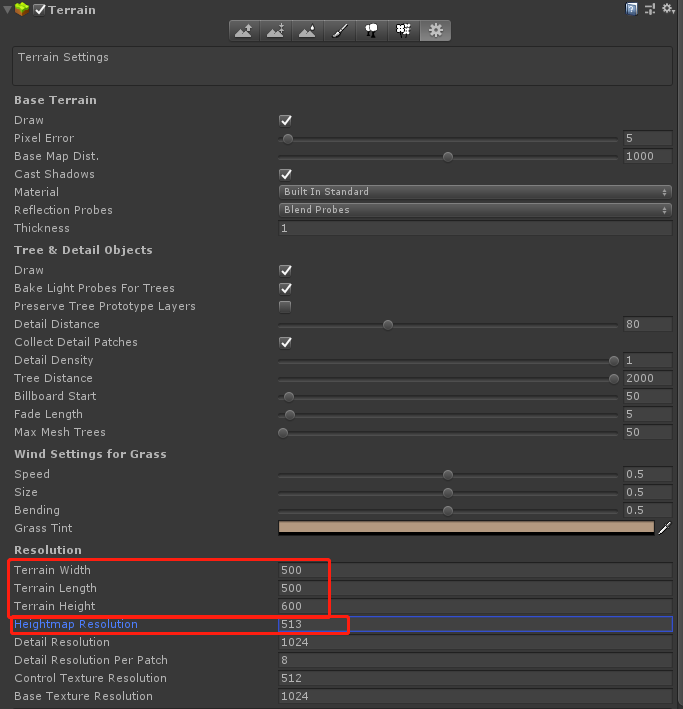这里提下Heightmap Resolution为啥是513：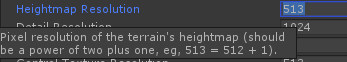float[,,] alphaMapData = terrainData.GetAlphamaps(0, 0, terrainData.alphamapWidth, terrainData.alphamapHeight);

这个函数的意思是，返回从(0,0)开始，到(terrainData.alphamapWidth, terrainData.alphamapHeight)宽度和高度的三维数组。
float[,]第一维和第二维构成了对应(x,y)坐标；第三维是对应SplatAlpha贴图的哪个通道，而float[,]的值，是对应通道的值。
比如我们自己创建的地形使用到两个贴图，那么只有一个splatalpha，unity使用RGBA四个通道值，对应四个贴图的混合度。如下图所示你可能看得更明白一点：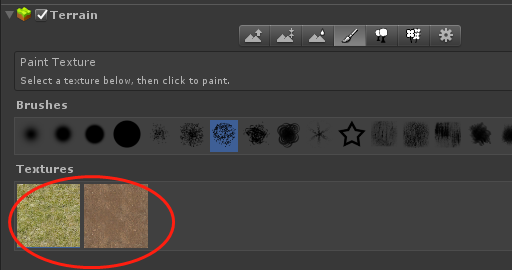这里使用两个贴图，所以根绝RGBA四个通道，只使用了RG通道。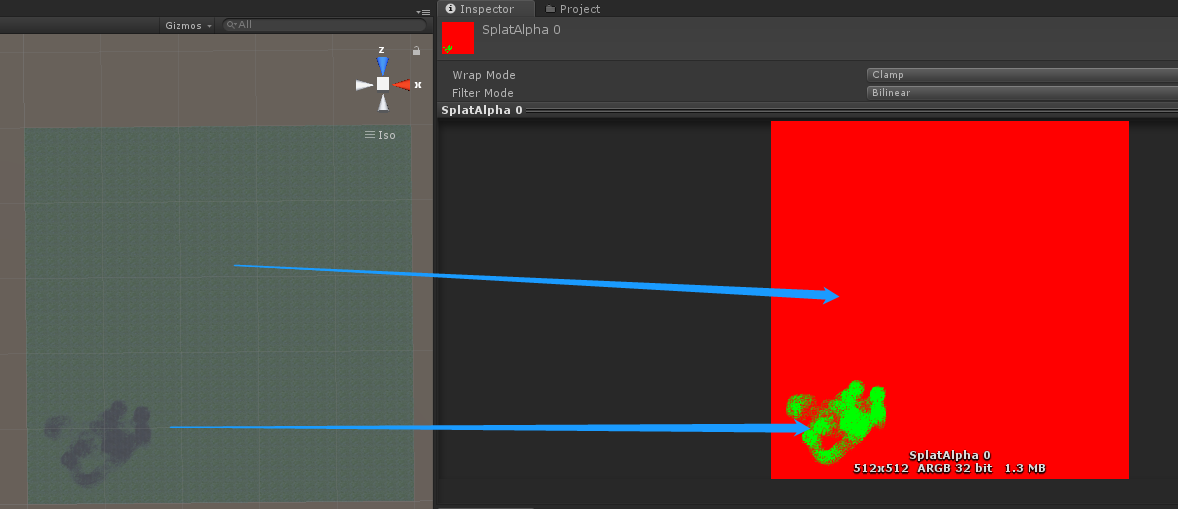第一个贴图对应的全是红色；第二个贴图对应的全是绿色。
如果有人问，如果是大于4个贴图怎么办，是的，会形成多个splatalpha贴图，这里只考虑最多四个贴图的情况。
我们可以使用如下的代码测试：
float aaaa = alphaMapData[0, 0, 0]; //第一个贴图的alpha值
float bbbb = alphaMapData[0, 0, 1]; //第二个贴图的alpha值

Vector3 meshScale = new Vector3(size.x / (w - 1f) * vertexCountScale, 1, size.z / (h - 1f) * vertexCountScale);

有人可能不太明白这个代码的意思，下面我就来具体的阐述一下：
如果要看懂的话，还需仔细阅下面的代码。
首先要确定点的x和y，然后确定z。那么到底要生成多少个点呢？
我们是根据高度图来生成mesh网格的。高度图的意思是对应(x,y)处的高度是多少。
有人就问了，难道地形是10001000，高度图不是10001000吗？可以是也可以不是，他们没有必然相等的要求，高度图可以低分辨率，比如上面的513，其实是512分辨率。
我的理解是地形是10001000，但是并不是所有的点都能对应一个高度图上的一个点，可以是多个点对应高度图上的同一个点。
下面我们就要理解下地形的长宽的意义了：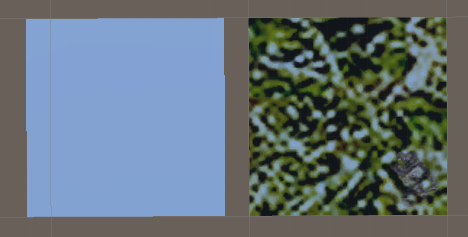下面我们就来确定下，到底要生成多少个点，以谁为标准生成点。
由于我们要确定点的高度，也就是y轴坐标，所以我们必须以高度图为标准生成点。那么我们就要在高度图上每4个点采样一个点，然后得到对应的height，组成(x,y,z)一个点。
那么有人会问了，如果地形是10241024，而高度图是512512，那么以高度图为标准，也就是生成了512/4=128
128128个点，也就是128128的地形，那么这个缩小了呀，本来是1024*1024大小的地形，这样就缩小了。
所以要有一个缩放的关系：
所以要求出本来地形的宽度和高度图宽度的几倍，然后再乘以每隔多少采样一个点，这样求出一个缩放系数，最后求出的点再乘以和这个系数，就恢复到了原来地形的尺寸了。
比如上面：地形10241024
高度图是：512512
所以1024/512=2
再者，每个4个单位取高度图，所以2*4=8
这样求出坐标之后，再乘以8即可。
代码如下：
int vertexCountScale = 4;
int w = terrainData.heightmapWidth; //高度图的宽度
int h = terrainData.heightmapHeight;//高度图的高度
Vector3 size = terrainData.size; //地形的长宽高
Vector3 meshScale = new Vector3(size.x / (w - 1f) * vertexCountScale, 1, size.z / (h - 1f) * vertexCountScale);

w = (w - 1) / vertexCountScale + 1;
h = (h - 1) / vertexCountScale + 1;

Vector3[] vertices = new Vector3[w * h]; //最后生成w*h这么多个点
for (int i = 0; i < w; i++)
{
for (int j = 0; j < h; j++)
{
int index = j * w + i;
float z = terrainData.GetHeight(i * vertexCountScale, j * vertexCountScale); //采样高度图
vertices[index] = Vector3.Scale(new Vector3(i, z, j), meshScale); //缩放会原来的地形尺寸
}
}

同理我们uv怎么计算呢？
如果要搞清楚uv是怎么计算的，就需要明白贴图的size以及offset是什么意思？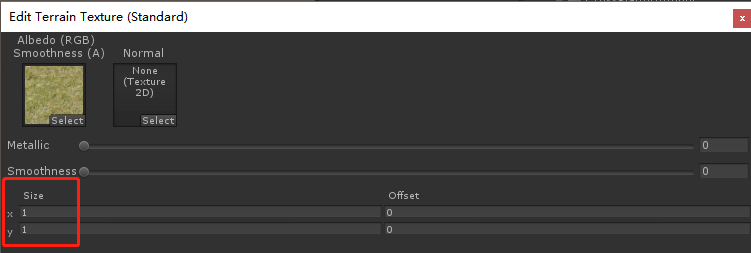可以看到这里的size，其实并不是我们通常意义的tile，它的意思就是size，就是定义了这个贴图的尺寸。那么地图被平铺多少个呢？使用地形的size.x/这里贴图的size的x即可。
而且每个贴图的wrapmode都是设置为repeat的方式：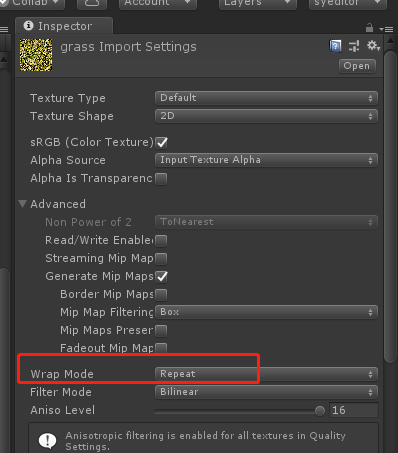Vector2 uvScale = new Vector2(1f / (w - 1f), 1f / (h - 1f)) * vertexCountScale *
(size.x /terrainData.splatPrototypes.tileSize.x);

new Vector2(1f / (w - 1f), 1f / (h - 1f))我们可以理解其实就是高度图的1/(w-1)以及1/(h-1)。
乘以vertexCountScale则是间隔多少的倍数。
而size.x/terrainData.splatPrototypes.tileSize.x，则是上面设置贴图里的size，也就是用地形的x除以这里的贴图的x，得到了水平平铺的倍数。
举例：
地形是88大小
高度图44大小
贴图是22大小
那么则要用贴图平铺44个贴图。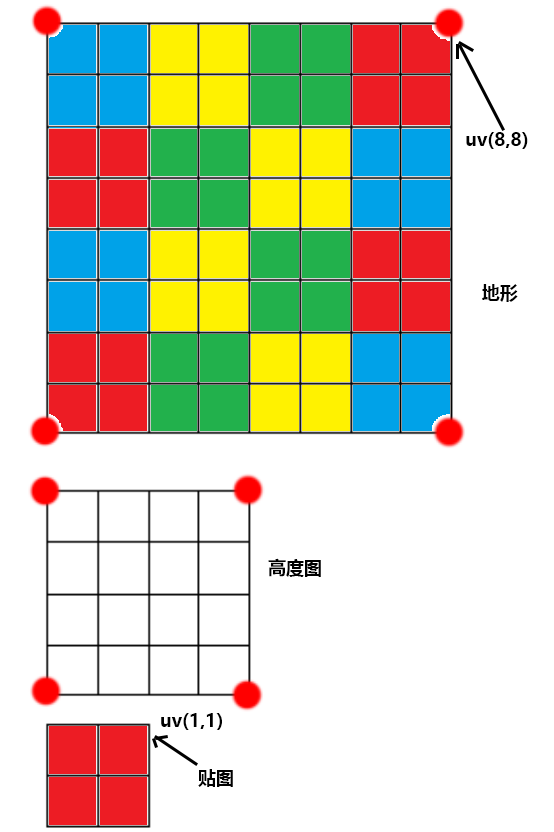所以完整的代码是：
Vector3 meshScale = new Vector3(size.x / (w - 1f) * vertexCountScale, 1, size.z / (h - 1f) * vertexCountScale);

Vector2 uvScale = new Vector2(1f / (w - 1f), 1f / (h - 1f))
* vertexCountScale * (size.x / terrainData.splatPrototypes.tileSize.x);     // [dev] 此处有问题，若每个图片大小不一，则出问题。日后改善

w = (w - 1) / vertexCountScale + 1;
h = (h - 1) / vertexCountScale + 1;

Vector3[] vertices = new Vector3[w * h];
Vector2[] uvs = new Vector2[w * h];
Vector4[] alphasWeight = new Vector4[w * h];

for (int i = 0; i < w; i++)
{
for (int j = 0; j < h; j++)
{
int index = j * w + i;
float z = terrainData.GetHeight(i * vertexCountScale, j * vertexCountScale);

Vector3 dddd = Vector3.Scale(new Vector3(i, z, j), meshScale);

vertices[index] = Vector3.Scale(new Vector3(i, z, j), meshScale);
uvs[index] = Vector2.Scale(new Vector2(i, j), uvScale);

// alpha map
int i2 = (int)(i * terrainData.alphamapWidth / (w - 1f));
int j2 = (int)(j * terrainData.alphamapHeight / (h - 1f));
i2 = Mathf.Min(terrainData.alphamapWidth - 1, i2);
j2 = Mathf.Min(terrainData.alphamapHeight - 1, j2);
var alpha0 = alphaMapData[j2, i2, 0];
var alpha1 = alphaMapData[j2, i2, 1];
//var alpha2 = alphaMapData[j2, i2, 2];
//var alpha3 = alphaMapData[j2, i2, 3];
alphasWeight[index] = new Vector4(alpha0, alpha1, 0, 0);
}
}

这里没有考虑到，每个贴图的size如果不一样的情况，所以为了简化纹理，可以将四个贴图的size设置为一样。
下面就是组织三角形网格了：
 /*
* 三角形
*     b       c
*      *******
*      *   * *
*      * *   *
*      *******
*     a       d
*/
int[] triangles = new int[(w - 1) * (h - 1) * 6];
int triangleIndex = 0;
for (int i = 0; i < w - 1; i++)
{
for (int j = 0; j < h - 1; j++)
{
int a = j * w + i;
int b = (j + 1) * w + i;
int c = (j + 1) * w + i + 1;
int d = j * w + i + 1;

triangles[triangleIndex++] = a;
triangles[triangleIndex++] = b;
triangles[triangleIndex++] = c;

triangles[triangleIndex++] = a;
triangles[triangleIndex++] = c;
triangles[triangleIndex++] = d;
}
}

不解释了，很容易。
同样我们还注意到，这里只有顶点信息、uv信息、三角形网格、alpha权重、但是没有法线信息，这个在这个博客中讲到：
https://blog.csdn.net/zr339361504/article/details/53352800

生成地形网格，我们需要保存顶点数据、瓦片贴图权重、瓦片uv坐标、光照贴图uv坐标、三角面、以及。由于与地形的光照部分直接使用烘培贴图，所以法线可以不用记录。

我们也可以计算出每个顶点的法线：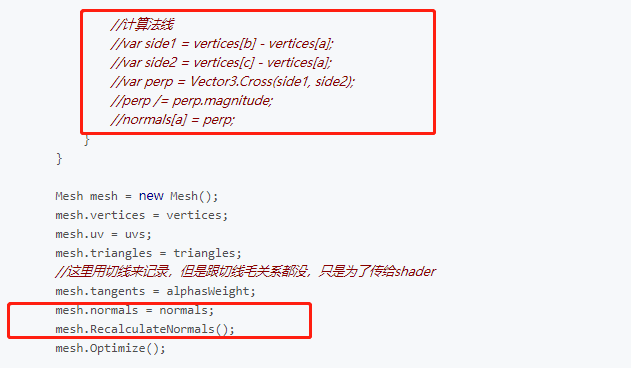这个地方存疑点？到底是怎么计算法线，还需要研究下。
 		Mesh mesh = new Mesh();
mesh.vertices = vertices;
mesh.uv = uvs;
mesh.triangles = triangles;
mesh.tangents = alphasWeight;       // 将地形纹理的比重写入到切线中

string transName = "[dev]MeshFromTerrainData";
var t = terrainObj.transform.parent.Find(transName);
if (t == null)
{
GameObject go = new GameObject(transName, typeof(MeshFilter), typeof(MeshRenderer), typeof(MeshCollider));
t = go.transform;
}

// 地形渲染
MeshRenderer mr = t.GetComponent<MeshRenderer>();
Material mat = mr.sharedMaterial;
if (!mat)

for (int i = 0; i < terrainData.splatPrototypes.Length; i++)
{
var sp = terrainData.splatPrototypes[i];
mat.SetTexture("_Texture" + i, sp.texture);
}

t.parent = terrainObj.transform.parent;
t.position = terrainObj.transform.position;
t.gameObject.layer = terrainObj.layer;
t.GetComponent<MeshFilter>().sharedMesh = mesh;
t.GetComponent<MeshCollider>().sharedMesh = mesh;
mr.sharedMaterial = mat;

t.gameObject.SetActive(true);
terrainObj.SetActive(false);

Debug.Log("Convert terrain to mesh finished!");

这样我们就根据terrain高度图，将terrain转换成mesh网格了。
Shader "Custom/Environment/TerrainSimple"
{
Properties
{
_Texture0 ("Texture 1", 2D) = "white" {}
_Texture1 ("Texture 2", 2D) = "white" {}
_Texture2 ("Texture 3", 2D) = "white" {}
_Texture3 ("Texture 4", 2D) = "white" {}
}

{
Tags { "RenderType" = "Opaque" }
LOD 200

Pass
{
CGPROGRAM
#pragma vertex vert
#pragma fragment frag

sampler2D _Texture0;
sampler2D _Texture1;
sampler2D _Texture2;
sampler2D _Texture3;

struct appdata
{
float4 vertex : POSITION;
float2 uv : TEXCOORD0;
float4 tangent : TANGENT;
};

struct v2f
{
float4 pos : SV_POSITION;
float2 uv : TEXCOORD0;
float4 weight : TEXCOORD1;
};

v2f vert(appdata v)
{
v2f o;
o.pos = UnityObjectToClipPos(v.vertex);
o.weight = v.tangent;
o.uv = v.uv;
return o;
}

fixed4 frag(v2f i) : SV_TARGET
{
fixed4 t0 = tex2D(_Texture0, i.uv);
fixed4 t1 = tex2D(_Texture1, i.uv);
fixed4 t2 = tex2D(_Texture2, i.uv);
fixed4 t3 = tex2D(_Texture3, i.uv);
fixed4 tex = t0 * i.weight.x + t1 * i.weight.y + t2 * i.weight.z + t3 * i.weight.w;
return tex;
}

ENDCG
}
}

Fallback "Diffuse"
}

Custom/Environment/TerrainSimple

这里是不支持光照计算的，也不支持光照贴图，所以完整的还需要参考：
http://www.cnblogs.com/jietian331/p/5831062.html
https://blog.csdn.net/zr339361504/article/details/53352800
但是http://www.cnblogs.com/jietian331/p/5831062.html 没有讲到法线的计算方式。
而https://blog.csdn.net/zr339361504/article/details/53352800讲到法线的计算方式，以及光照贴图的uv2计算。
两者的正确与否还是值得商榷的，但是这两篇也是唯一能够讲述怎么转terrain为mesh的博客了，如果有人知道其他的资料，麻烦留言给我，谢谢。
以上就是关于如果将unity自带的terrain转换为mesh的全部介绍了，下面我们将学习如果将mesh进行分割为小的mesh，并制定策略进行加载。


展开全文unity 地形
• 首先看一下最终效果:这里我假设读者已经了解并知道如何使用Mesh。如果不懂的话可以百度一下，有很多文章。实现起来其实非常简单，思路：每两个顶点为一组，这两个点的x坐标相同，一个在上一个在下，下边的y坐标固定...
首先看一下最终效果: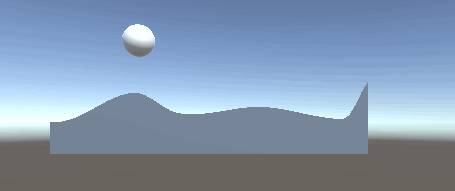这里我假设读者已经了解并知道如何使用Mesh。如果不懂的话可以百度一下，有很多文章。实现起来其实非常简单，思路：每两个顶点为一组，这两个点的x坐标相同，一个在上一个在下，下边的y坐标固定为0，上边的y坐标从曲线编辑器中取值。然后把生成的mesh赋予物体的MeshCollider。下面给出完整代码:using System.Collections;
using System.Collections.Generic;
using UnityEngine;

public class MeshMount : MonoBehaviour {
//每2个定点为一组，此值代表有多少组
const int count = 50;
//每两组顶点的间隔距离，此值越小曲线越平滑
const float pointdis = 0.2f;

Material mat;
MeshCollider mc;

//曲线
public AnimationCurve anim;

// Use this for initialization
void Start () {

mr.material = mat;

DrawSquare();
}

// Update is called once per frame
void Update () {

}

void DrawSquare()
{
//创建mesh
mesh.Clear();

//定义顶点列表
List<Vector3> pointList = new List<Vector3>();
//uv列表
List<Vector2> uvList = new List<Vector2>();
//第一列的前2个点直接初始化好

//设置前2个点的uv

//三角形数组
List<int> triangleList = new List<int>();

for (int i = 0; i < count; i ++){
//计算当前列位于什么位置
float rate = (float)i / (float)count;
//计算当前的顶点
pointList.Add(new Vector3((i + 1)*pointdis, 0, 0));

//这里从曲线函数中取出当点的高度

//uv直接使用rate即可

//计算当前2个点与前面2个点组成的2个三角形
int startindex = i * 2;

}

//把最终的顶点和三角形数组赋予mesh;
mesh.vertices = pointList.ToArray();
mesh.triangles = triangleList.ToArray();
mesh.uv = uvList.ToArray();
mesh.RecalculateNormals();

//把mesh赋予MeshCollider
mc.sharedMesh = mesh;
}
}
使用方法：首先创建一个空物体，把脚本挂到此物体上，然后在Inspector面板中双击anmi，编辑出一个喜欢的曲线形状。注意横轴只有在[0,1]的范围内才有效。接下来创建一个球体并赋予rigidbode。最后运行场景就能看到文章最开始的效果了。
展开全文untiy
• Unity3D动态创建地形网格（一） 这次简单的写一个动态创建地形网格的脚本给大家分享一下。 这次是第一部分，仅仅实现了通过高度图动态生成地形的部分。假如以后有心情和时间，再来慢慢的补充多通道刷地形材质、...unity
• 转载：http://www.cnblogs.com/kyokuhuang/p/4191169.htmlunity3d
• 一、创建一个GameObject,并在上面挂两个组件(MeshFilter、MeshRenderer) 二、新建个脚本，并挂在刚才创建的GameObject上  using UnityEngine; using System.Collections;  public class ...
• 之前写过一篇博客，当时主要介绍的自绘地形并创建地形，着重介绍顶点排序处理以及分割三角形问题。当时由于着急，忽略了一些问题。之前写的创建mesh其实是有问题的，我只给mesh赋上顶点信息，并没有赋上发现信息以及...unity3d
• Mesh概念：MeshUnity中的一个组件，称为网格组件。通俗的讲，Mesh是指模型的网格，3D模型是由多边形拼接而成，而多边形实际上是由多个三角形拼接而成的。所以一个3D模型的表面其实是由多个彼此相连的三角面构成。...
• Unity3d_实现地形上挖洞 Unity3d_实现地形上挖洞 Unity3d_实现地形上挖洞Unity3d
•unity3d
• 插件下载：https://assetstore.unity.com/packages/tools/terrain/terrain-to-mesh-47276?aid=1011lGkb&utm_source=aff
• 用于将使用3dmax，Terragen或任何其他编辑器创建的3D地形模型快速转换为Unity Terrain的组件。 特征： •支持Unity v5.2 - Unity 2018.x •组件中使用的模型和地形数量无限; •地形在网格的相同位置创建; •将模型的...
• 在游戏开发的制作中可能需要制作地形，需要制作的相关的地形工具，绝大部分情况下并不会使用unity的terrain，因为其在移动平台上的表现很差，相同顶点甚至更多的mesh性能也比其优秀很多（或许是因为其内部动态生成...unity
• 一、首先创建 Terrain 二、调整 terrain 合适的大小，并移动到合适的位置三、打开 Mesh To Terrain 面板四、将 FBX 地形 拖到 GameObjects 上，将Terrain 拖到 Terrain上，点击 Start，就可以创建简单的 Unity3d ...Unity3D
•unity3dUnity